# = 2. We wish to 7. Consider the differential equation y' + y = 2.. with...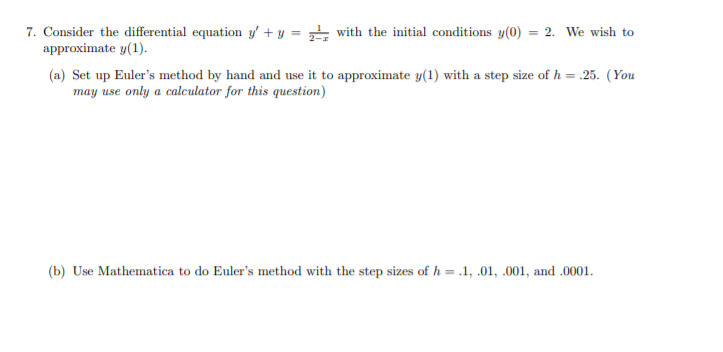= 2. We wish to 7. Consider the differential equation y' + y = 2.. with the initial conditions y(0) approximate y(1). (a) Set up Euler's method by hand and use it to approximate y(1) with a step size of h = 25. (You may use only a calculator for this question) (b) Use Mathematica to do Euler's method with the step sizes of h= .1,.01, .001, and .0001.

ANSSWER :

Consider the differential equation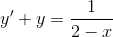with the inital conditions y(0) =2 we wish to approximate y(1).

Didfferntial equation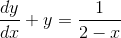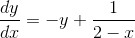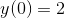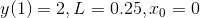Apply Euler's Method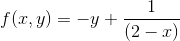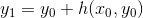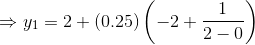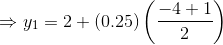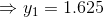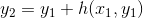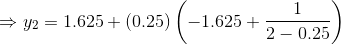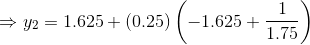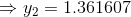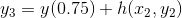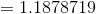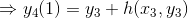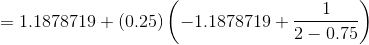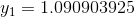b)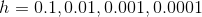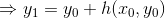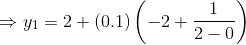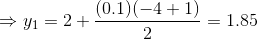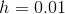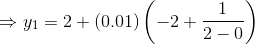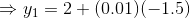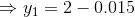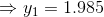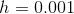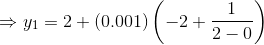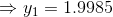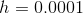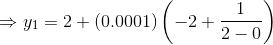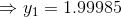##### Add Answer of: = 2. We wish to 7. Consider the differential equation y' + y = 2.. with...
Similar Homework Help Questions
• ### e differential equation y 0 + y = 1 2−x with the initial conditions y(0) =...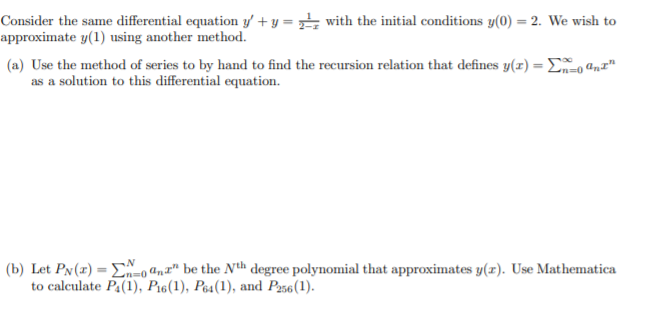e differential equation y 0 + y = 1 2−x with the initial conditions y(0) = 2. We wish to approximate y(1) using another method. please help me, thanks so much Consider the same differential equation y' +y= with the initial conditions y(0) = 2. We wish to approximate y(1) using another method. (a) Use the method of series to by hand to find the recursion relation that defines y(t) = 2*, QmI" as a solution to this differential equation....

• ### Please teach me this.. Consider the same differential equation y' +y= with the initial conditions y(0)...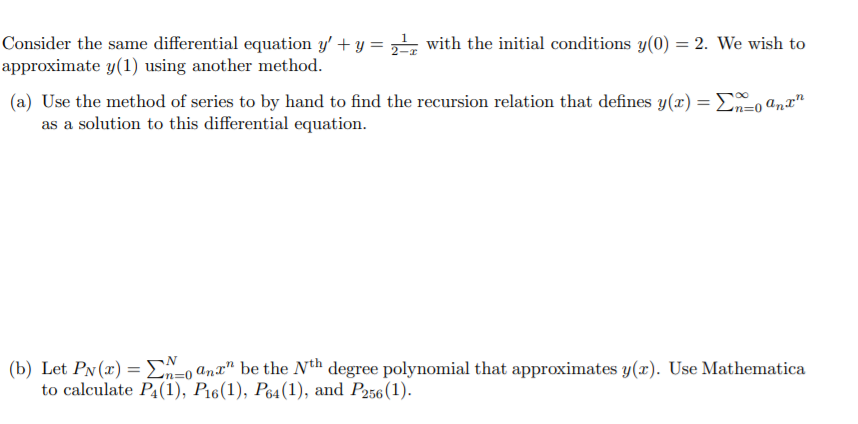Please teach me this.. Consider the same differential equation y' +y= with the initial conditions y(0) = 2. We wish to approximate y(1) using another method. (a) Use the method of series to by hand to find the recursion relation that defines y(t) = {mo anx" as a solution to this differential equation. (b) Let Pn(x) = EX=anx" be the Nth degree polynomial that approximates y(x). Use Mathematica to calculate P4(1), P16(1), P64(1), and P256 (1).

• ### 3. Euler's Method (a) Use Euler's Method with step size At = 1 to approximate values...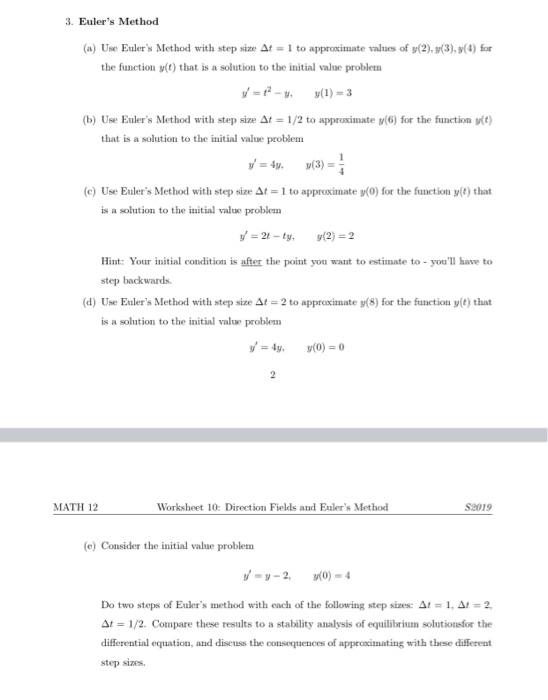3. Euler's Method (a) Use Euler's Method with step size At = 1 to approximate values of y(2),3(3), 3(1) for the function y(t) that is a solution to the initial value problem y = 12 - y(1) = 3 (b) Use Euler's Method with step size At = 1/2 to approximate y(6) for the function y(t) that is a solution to the initial value problem y = 4y (3) (c) Use Euler's Method with step size At = 1 to...

• ### Using MATLAB_R2017a, solve #3 using the differential equation in question #2 using Simulink, pres...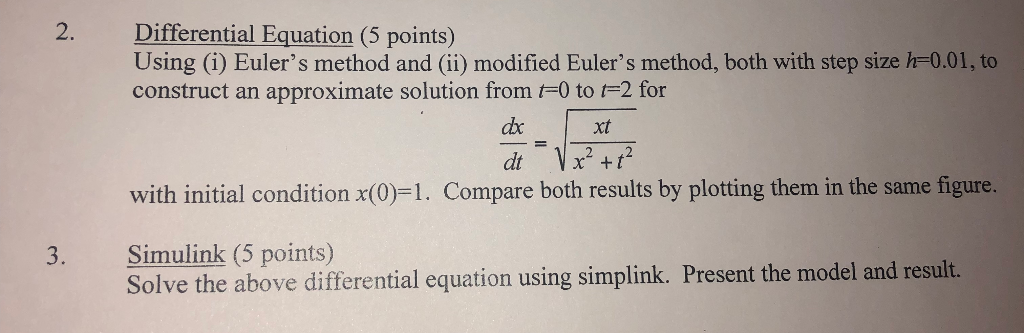Using MATLAB_R2017a, solve #3 using the differential equation in question #2 using Simulink, present the model and result. 2. Differential Equation (5 points) Using (i) Euler's method and (ii) modified Euler's method, both with step size h-0.01, to construct an approximate solution from F0 to F2 for xt 2, 42 with initial condition x(0)=1. Compare both results by plotting them in the same figure. 3. Simulink (5 points) Solve the above differential equation using simplink. Present the model and result....

• ### 2. Differential Equation (5 points) Using (i) Euler's method and (ii) modified Euler's method, bo...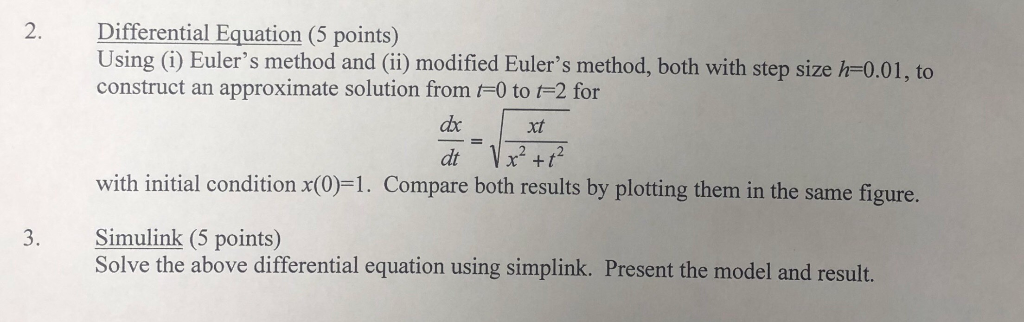Please show Matlab code and Simulink screenshots 2. Differential Equation (5 points) Using (i) Euler's method and (ii) modified Euler's method, both with step size h-0.01, to construct an approximate solution from t-0 to t-2 for xt 2 , 42 with initial condition x(0)-1. Compare both results by plotting them in the same figure. 3. Simulink (5 points) Solve the above differential equation using simplink. Present the model and result. 2. Differential Equation (5 points) Using (i) Euler's method and...

• ### Problem Thre: 125 points) Consider the following initial value problem: dy-2y+ t The y(0) -1 ea dt ical solution of the differential equation is: y(O)(2-2t+3e-2+1)y fr exoc the differential equat...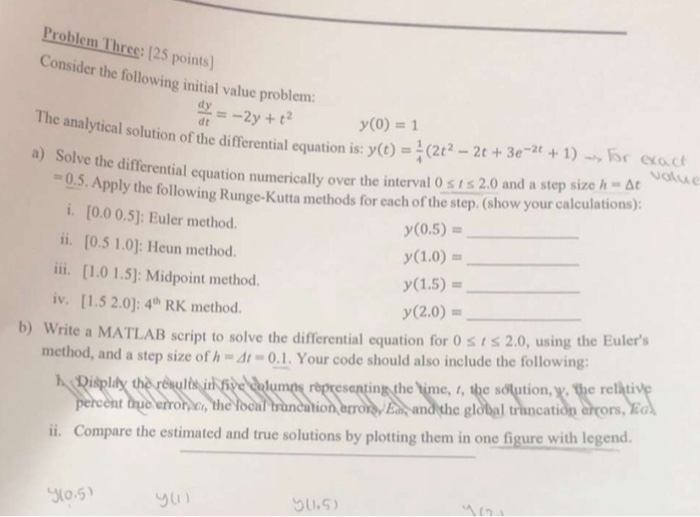Problem Thre: 125 points) Consider the following initial value problem: dy-2y+ t The y(0) -1 ea dt ical solution of the differential equation is: y(O)(2-2t+3e-2+1)y fr exoc the differential equation numerically over the interval 0 s i s 2.0 and a step size h At 0.5.A Apply the following Runge-Kutta methods for each of the step. (show your calculations) i. [0.0 0.5: Euler method ii. [0.5 1.0]: Heun method. ii. [1.0 1.5): Midpoint method. iv. [1.5 2.0): 4h RK method...

• ### (d) This part of question is concerned the use the Euler's method to solve the following initial-...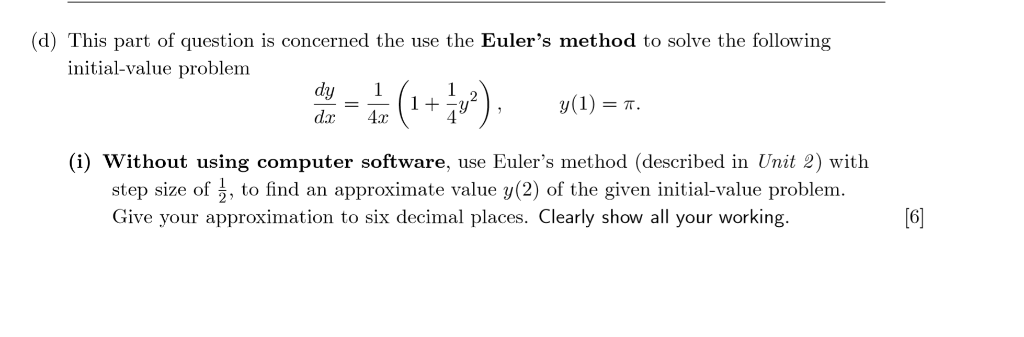(d) This part of question is concerned the use the Euler's method to solve the following initial-value problem dy dx4ar (i) Without using computer software, use Euler's method (described in Unit 2) with step size of 2, to find an approximate value y(2) of the given initial-value problem. Give your approximation to six decimal places. Clearly show all your working 6 (ii) Use Mathcad worksheet Έυ1er's method, associated with Unit 2 to computer the MATHCAD estimate solutions and absolute errors...

• ### 1. Consider the following differential equation. ag = ty, y(0)=1. dt (a) Use Euler's Method with...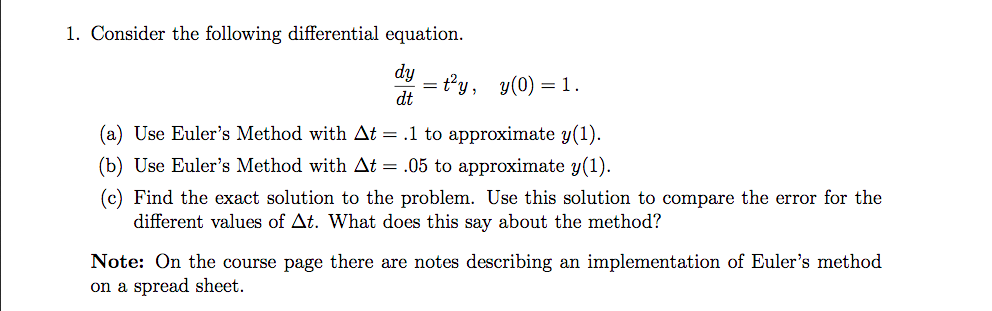1. Consider the following differential equation. ag = ty, y(0)=1. dt (a) Use Euler's Method with At = .1 to approximate y(1). (b) Use Euler's Method with At = .05 to approximate y(1). (c) Find the exact solution to the problem. Use this solution to compare the error for the different values of At. What does this say about the method? Note: On the course page there are notes describing an implementation of Euler's method on a spread sheet.

• ### 2. Now let's investigate how the various methods work when applied to an especially simple differential equation, x...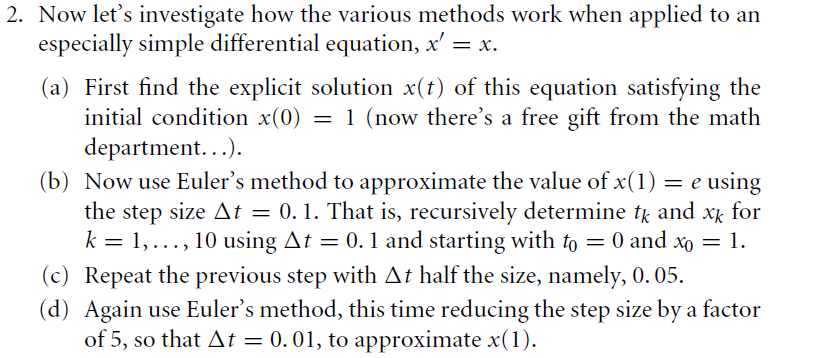2. Now let's investigate how the various methods work when applied to an especially simple differential equation, x' x (a) First find the explicit solution x(t) of this equation satisfying the initial condition x(0) = 1 (now there's a free gift from the math department... (b) Now use Euler's method to approximate the value of x(1)e using the step size At = 0.1. That is, recursively determine tk and xk for k 1,.., 10 using At = 0.1 and starting...

• ### MATLAB CODE: Task 2 8y dt Solve the above ordinary differential equation (ODE) using Euler's method with step sizes of: 2. h 0.75 3. h 0.5 4. h 0.001 a) For each step size, plot the results at eac...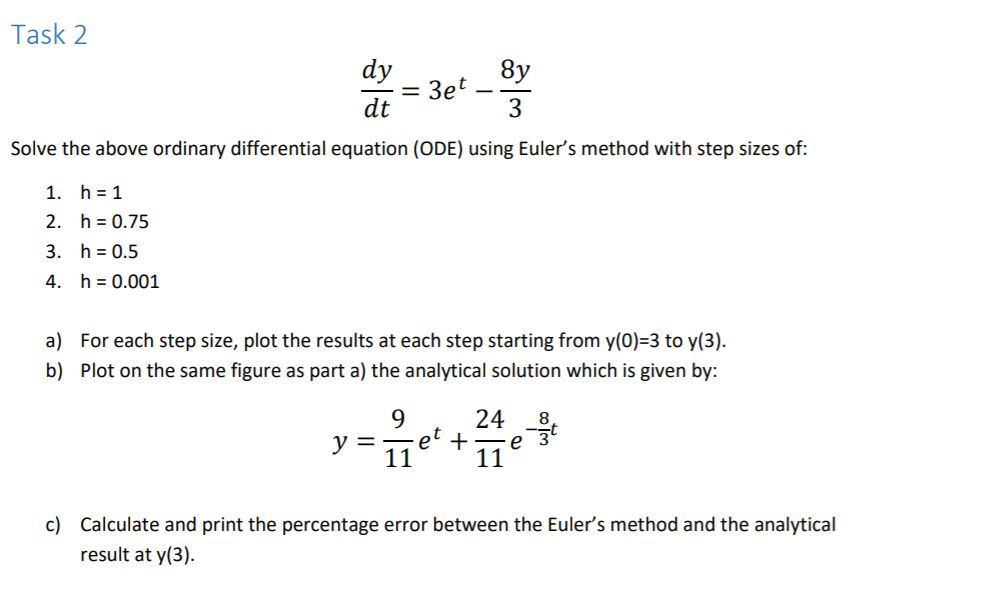MATLAB CODE: Task 2 8y dt Solve the above ordinary differential equation (ODE) using Euler's method with step sizes of: 2. h 0.75 3. h 0.5 4. h 0.001 a) For each step size, plot the results at each step starting from y(0) 3 to y(3). b) Plot on the same figure as part a) the analytical solution which is given by: 9 24 -8t c) Calculate and print the percentage error between the Euler's method and the analytical result...

Free Homework App